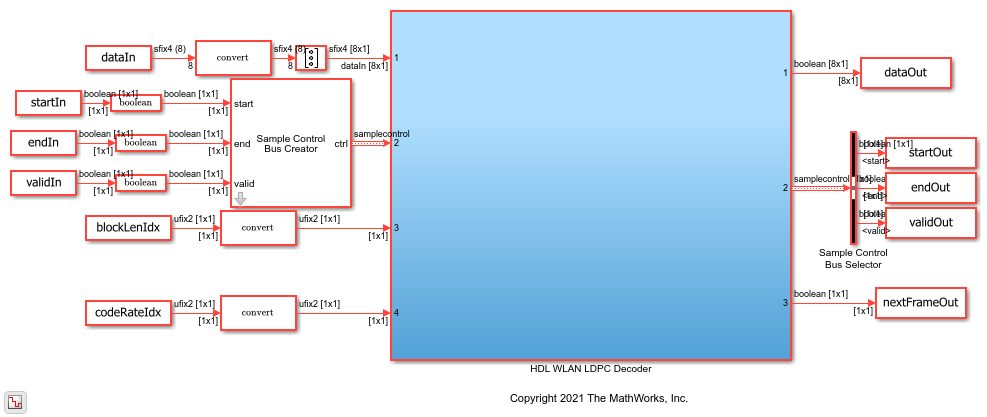# Decode WLAN LDPC Streaming Data

This example shows how to simulate the WLAN LDPC Decoder block and compare the hardware-optimized results with the results from the Communication Toolbox™ function.

Generate the input to the block using the Communication Toolbox function `ldpcEncode`. Provide the generated data as the input to the WLAN LDPC Decoder block and the Communication Toolbox function `ldpcDecode`. Compare the output of the block with the output of the `ldpcDecode` function. This example contains two Simulink® models. One model is configured to support the WLAN standards IEEE 802.11 n/ac/ax, and other model is configured to support the standard IEEE 802.11 ad. When you run the script, the respective model is selected based on the value that you specify for the variable `standard` mentioned in the script.

### Set Up Input Variables

Choose a series of input values for the block length and code rate according to the WLAN standard. You can change the variable values in this section based on your requirements.

```standard = 'IEEE 802.11 n/ac/ax'; % IEEE 802.11 n/ac/ax or IEEE 802.11 ad codeRateIdx = [0; 1; 2; 3]; % Code rate index blkLenIdx = [0; 1; 2; 0]; % Block length index numFrames = 4; scalar = false; % true for scalar inputs and false % for vector inputs algorithm = 'Min-sum'; % Min-sum or Normalized min-sum niter = 8; % Number of iterations if strcmpi(algorithm,'Min-sum') alpha = 1; else alpha = 0.75; % Scaling factor, which must be in % the range [0.5:0.0625:1] end ```

### Generate Input Data

Generate inputs for the `ldpcEncode` function with the specified block length and code rate variables. Use the encoded data from the `ldpcEncode` function, modulate the data using a modulator function, add noise using a channel System object™, and generate log-likelihood ratio (LLR) values using a symbol demodulator function. After that, provide these LLR values as an input to the `ldpcDecode` function.

Create vectors of block length index and code rate index using the `blockLenIdx` and `codeRateIdx` variables, respectively. Convert the frames of LLR values to samples with a control bus signal that indicates the frame boundaries. Provide these vectors and control bus as an input to the WLAN LDPC Decoder block.

The `decFrameGap` variable in the script accommodates the latency of the WLAN LDPC Decoder block for the specified block length, code rate, and number of iterations. Use the nextFrame output signal to determine when the block is ready to accept the start of the next input frame.

```% Initialize inputs msg = {numFrames}; % Input to |ldpcEncode| function rxLLR = cell(1,numFrames); % Input to |ldpcDecode| function refOut = cell(1,numFrames); % Output of |ldpcDecode| function decSampleIn = []; decStartIn = []; decEndIn = []; decValidIn = []; decBlkLenIdxIn = []; decCodeRateIdxIn = []; for ii = 1:numFrames if strcmpi(standard,'IEEE 802.11 n/ac/ax') blockLenSet = [648,1296,1944]; rateSet = {'1/2','2/3','3/4','5/6'}; blkLen = blockLenSet(blkLenIdx(ii)+1); codeRate = rateSet{codeRateIdx(ii)+1}; modelName = 'HDLWLANLDPCDecoderStd11ac'; else rateSet = {'1/2','5/8','3/4','13/16'}; blkLen = 672; codeRate = rateSet{codeRateIdx(ii)+1}; modelName = 'HDLWLANLDPCDecoderStd11ad'; end [rxLLR{ii},refOut{ii},msg{ii}] = inputGenForWLANLDPCDec(blkLen,codeRate,niter,alpha); if scalar decFrameGap = niter*1000 + length(msg{ii}); %#ok vecSize = 1; len = length(rxLLR{ii}); else len = length(rxLLR{ii})/8; vecSize = 8; decFrameGap = niter*1000 + ceil(length(msg{ii})/8); end decIn = reshape(rxLLR{ii},vecSize,[]); decSampleIn = [decSampleIn decIn zeros(size(decIn,1),decFrameGap)]; %#ok<*AGROW> decStartIn = logical([decStartIn 1 zeros(1,len-1) zeros(1,decFrameGap)]); decEndIn = logical([decEndIn zeros(1,len-1) 1 zeros(1,decFrameGap)]); decValidIn = logical([decValidIn ones(1,len) zeros(1,decFrameGap)]); decBlkLenIdxIn = ([decBlkLenIdxIn repmat(blkLenIdx(ii),1,len) zeros(1,decFrameGap)]); decCodeRateIdxIn = ([decCodeRateIdxIn repmat(codeRateIdx(ii),1,len) zeros(1,decFrameGap)]); end dataIn = timeseries(fi(decSampleIn',1,4,0)); startIn = timeseries(decStartIn); endIn = timeseries(decEndIn); validIn = timeseries(decValidIn); if strcmpi(standard,'IEEE 802.11 n/ac/ax') blockLenIdx = timeseries(fi(decBlkLenIdxIn,0,2,0)); end codeRateIdx = timeseries(fi(decCodeRateIdxIn,0,2,0)); % For the standard % IEEE 802.11 ad simTime = length(decValidIn); ```

The `HDL WLAN LDPC Decoder` subsystem contains the WLAN LDPC Decoder block. Running the model imports the input signal variables `dataIn`, `startIn`, `endIn`, `validIn`, `blockLenIdx`, `codeRateIdx`, `niter`, and `simTime` to the block from the script and exports a stream of decoded output samples `dataOut` and a control bus containing `startOut`, `endOut`, and `validOut` signals from the block to the MATLAB workspace.

```open_system(modelName); if alpha ~= 1 set_param([modelName '/HDL WLAN LDPC Decoder/WLAN LDPC Decoder'], ... 'Algorithm','Normalized min-sum'); set_param([modelName '/HDL WLAN LDPC Decoder/WLAN LDPC Decoder'], ... 'ScalingFactor',num2str(alpha)); else set_param([modelName '/HDL WLAN LDPC Decoder/WLAN LDPC Decoder'], ... 'Algorithm','Min-sum'); end sim(modelName); ```### Compare Simulink Block Output with MATLAB Function Output

Convert the streaming data output of the WLAN LDPC Decoder block to frames. Compare the frames with the output of the `ldpcDecode` function.

```startIdx = find(squeeze(startOut)); endIdx = find(squeeze(endOut)); dec = squeeze(dataOut); decHDL = {numFrames}; for ii = 1:numFrames idx = startIdx(ii):endIdx(ii); if scalar decHDL{ii} = dec(idx); else decHDL{ii} = dec(:,idx); end HDLOutput = decHDL{ii}(1:length(refOut{ii})); error = sum(abs(double(refOut{ii})-HDLOutput(:))); fprintf(['Decoded frame %d: Output data differs by %d bits\n'],ii,error); end ```
```Decoded frame 1: Output data differs by 0 bits Decoded frame 2: Output data differs by 0 bits Decoded frame 3: Output data differs by 0 bits Decoded frame 4: Output data differs by 0 bits ```